# Arc Of A Circle

Here we will learn about the arc of a circle, including how to find the arc length and work backwards given the arc length to solve problems.

There are also arc of a circle worksheets based on Edexcel, AQA and OCR exam questions, along with further guidance on where to go next if you’re still stuck.

## What is an arc of a circle?

An arc of a circle is a portion of the circumference of a circle.

In GCSE mathematics the arc of a circle can be used to define angles and lengths.

The arc of a circle would be the curved edge of a sector or segment.

There are circle theorems that are defined using arcs of the circle.

For example, \theta is the angle subtended by arc AC.

We can find the length of an arc if we know the angle of the sector.

### What is an arc of a circle?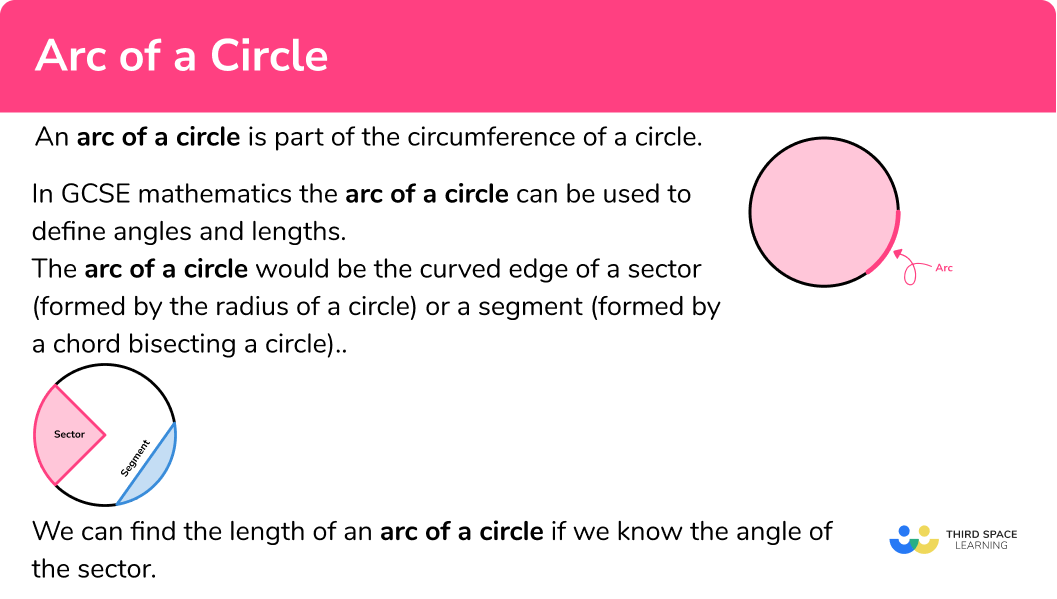### Calculating the arc length

The arc length is a fraction of the circumference of the circle.

The fraction will be \frac{\theta}{360}, if \theta is the angle of the sector.

The arc length formula is,

\text{Arc length } = \frac{\theta}{360} \times \pi\times d

\theta = \text{ angle of the sector}

d = \text{ diameter of the circle}

Or

\text{Arc length } = \frac{\theta}{360} \times 2\times\pi \times r

\theta = \text{ angle of the sector}

r = \text{ radius of the circle}

## How to calculate the arc length

In order to calculate the arc length:

1. Find the length of the radius.
2. Find the size of the angle creating the sector.
3. Substitute the values you know into the formula for the arc length.

### Explain how to calculate the arc length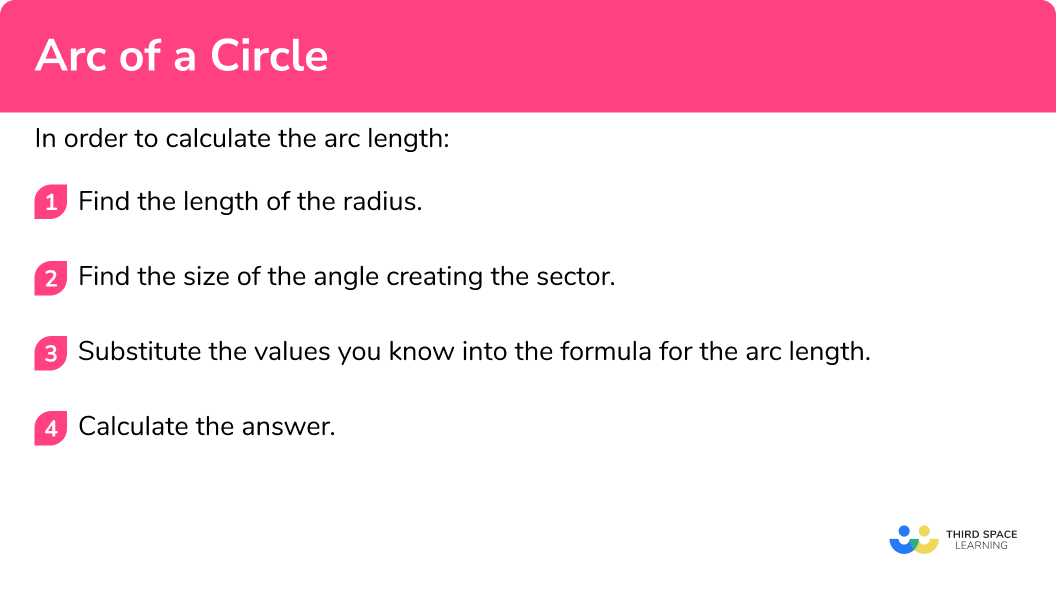Arc of a circle is part of our series of lessons to support revision on circles, sectors and arcs. You may find it helpful to start with the main circles, sectors and arcs lesson for a summary of what to expect, or use the step by step guides below for further detail on individual topics. Other lessons in this series include:

## Calculating the arc length examples

### Example 1: calculate the arc length given the angle

Calculate the arc length of the sector shown below.

1. Find the length of the radius/diameter.

2Find the size of the angle creating the sector.

Angle = 115^{\circ}

3Substitute the values you know into the formula for the arc length.

As you know the radius you can use the formula which has ‘r’ as a variable.

\begin{aligned} \text{Arc length } &=\frac{\theta}{360} \times 2\times\pi \times r \\\\ &=\frac{115}{360} \times 2\times\pi \times 8 \\\\ &=\frac{46}{9} \pi \end{aligned}

\begin{aligned} \text{Arc length } &=\frac{46}{9}\pi \ cm \\\\ &=16.0570...cm \end{aligned}

The arc measures =16.1 \ cm .

### Example 2: calculate the arc length not given the angle

Calculate the arc length of the sector shown below.

Although we can use the radius or diameter as long as we use the correct formula for each.

The angle creating the sector is the angle included between the two radii.

To find it in this case we need to use the angle fact, angles around a point add to 360^{\circ}.

360-240=120

The angle creating the sector is 120^{\circ}.

\begin{aligned} \text { Arc length }&=\frac{\theta}{360} \times 2 \times \pi \times r \\\\ &=\frac{120}{360} \times 2 \times \pi \times 4 \end{aligned}

\begin{aligned} &=8.37758041 \\\\ &=8.38 \mathrm{~m} \end{aligned}

### Example 3: finding the arc length not given the radius or angle

Below is a sector of a circle.

The diameter of the circle is 12 \ cm.

Calculate the arc length.

Diameter = 12 \ cm

The radius is half of the diameter, so the radius = 6 \ cm.

Although we can use the radius or diameter as long as we use the correct formula for each.

The angle creating the sector is the angle included between the two radii.

To find it in this case we need to use the angle fact, angles around a point add to 360^{\circ}.

360-205=155

The angle creating the sector is 155^{\circ}.

\begin{aligned} \text { Arc length }&=\frac{\theta}{360} \times 2 \times \pi \times r \\\\ &=\frac{155}{360} \times 2 \times \pi \times 6 \end{aligned}

\begin{aligned} &=16.23156204 \\\\ &=16.2 \mathrm{~cm} \end{aligned}

### Common misconceptions

• Finding the length of the circumference not the length of the arc

Remember to find the fraction of the circle that makes the arc not just the circumference of the whole circle.

For example,

Use \frac{\theta}{360} \times \pi\times d

or \frac{\theta}{360} \times 2\times\pi \times r .

Not \pi\times d

or 2\times\pi \times r.

• Incorrect angle unit set on calculator

At GCSE all angles are measured in degrees. Make sure that your calculator has a small ‘d’ for degrees at the top of the screen rather than an ‘r’ for radians – these are not used until A Level.

### Practice arc of a circle questions

1. What part of a circle does the red line show?SegmentMajor arcMinor arcSectorPart of the circumference is called an arc. The red line is more than half of the circumference, so it is a major arc.

2. The diagram shows a semicircle. What is the length of the curved edge, in terms of \pi.8 \pi \ cm8\pi+8 \ cm4\pi \ cm2 \pi \ cmThe length of the curved edge of a semicircle can be found by calculating \pi d \div 2.

3. Find the length of the arc of the sector. Give your answer to 1 decimal place.9.4 \ cm28.3 \ cm18.8 \ cm37.7 \ cmThe length of the curved edge of a quarter-circle can be found by calculating \pi d \div 4.

4. Find the length of the arc of the sector. Give your answer to 1 decimal place.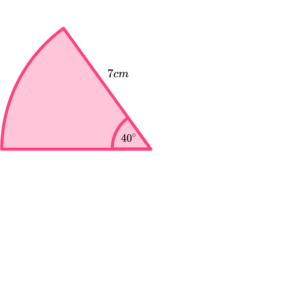2.4 \ cm7.3 \ cm17.1 \ cm4.9 \ cmSubstituting the values we know into the formula for the arc length,

\begin{aligned} \text{Arc length } &=\frac{\theta}{360} \times 2 \times \pi \times r \\\\ &=\frac{40}{360} \times 2 \times \pi \times 7 \\\\ &=4.886921906 \mathrm{~cm} \end{aligned}

5. Find the length of the arc of the sector. Give your answer to 1 decimal place.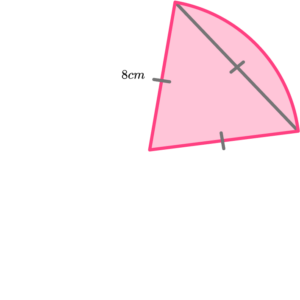16.8 \ cm4.2 \ cm8.4 \ cm12.6 \ cmSubstituting the values we know into the formula for the arc length, remembering \theta =60^{\circ} because this is an equilateral triangle.

\begin{aligned} \text{Arc length } &=\frac{\theta}{360} \times 2 \times \pi \times r \\\\ &=\frac{60}{360} \times 2 \times \pi \times 8 \\\\ &=8.37758041 \mathrm{~cm} \end{aligned}

6. Find the angle of the sector with an arc length of 10 \pi \ cm and a radius of 12 \ cm.

150^{\circ}210^{\circ}60^{\circ}30^{\circ}Substituting the values we know into the formula for arc length,

\frac{\theta}{360} \times 2 \times \pi \times 12=10 \pi

Solving for the angle \theta,

\theta=150^{\circ}.

### Arc of a circle GCSE questions

1. A sector has a radius of 15 \ cm and an angle of 120^{\circ}. Find the length of the arc in terms of \pi.

(2 marks)

Use of \frac{\theta}{360} \times \pi d .

(1)

10 \pi

(1)

2. The sector has an angle of 50^{\circ} and an arc length of 25 \ cm. Find the radius of the sector in centimetres to the nearest millimetre.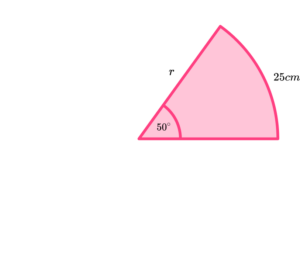(3 marks)

25=\frac{50}{360} \times \pi d (or equivalent).

(1)

Diameter = 57.29…

(1)

(1)

3. A metal sector with radius 6 \ cm and angle 250^{\circ} is used to form an open cone, as shown.

Calculate the radius, r, of the cone formed from the sector.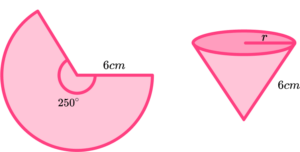(4 marks)

Use of \frac{250}{360} \times 12 \pi .

(1)

Arc length = 26.1799…

(1)

\pi r^{2}=26.1799…

(1)

r=2.89 \ cm

(1)

## Learning checklist

You have now learned how to:

• Identify and apply circle definitions and properties, including tangent, arc, sector and segment
• Calculate the length of an arc
• Calculate a property of a circle given the length of an arc

## Still stuck?

Prepare your KS4 students for maths GCSEs success with Third Space Learning. Weekly online one to one GCSE maths revision lessons delivered by expert maths tutors.

Find out more about our GCSE maths tuition programme.